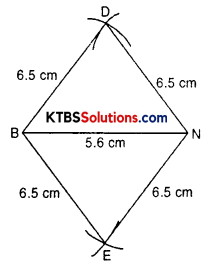# KSEEB Solutions for Class 8 Maths Chapter 4 Practical Geometry Ex 4.2

You can Download KSEEB Solutions for Class 8 Maths Chapter 4 Practical Geometry Ex 4.2 Questions and Answers helps you to revise the complete syllabus.

## KSEEB Solutions for Class 8 Maths Chapter 4 Practical Geometry Ex 4.2

Question 1.
LI = 4 cm
IF = 3 cm
TL = 2.5 cm
LF = 4.5 cm
IT = 4 cm

OL = 7.5 cm
GL = 6 cm
GD = 6 cm
ID = 5 cm
OD = 10 cm(iii) Rhombus BEND
BN = 5.6 cm
BD = 6.5 cm
Solution:
(i) Draw a rough sketch of quadrilateral LIFT.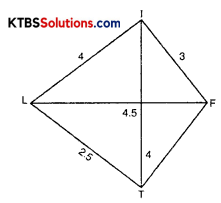Steps of construction:

1. Draw a line segment LF = 4.5 cm.
2. Draw an arc of radius 4 cm from point L.
3. Draw an arc of radius 3 cm from point F.
4. Both these arcs intersect, let it say point I.
5. LIF is a triangle.
6. Draw an arc of radius 4 cm from point I on the opposite side of LF.
7. Draw an arc of radius 2.5 cm from point L intersecting at T.
8. Join LT and TF, LIFT is a required quadrilateral.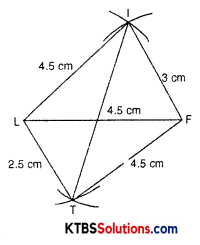(ii) Draw a rough sketch of quadrilateral GOLD.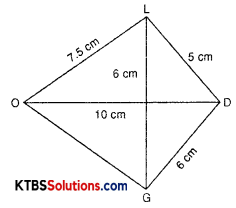Steps of construction:

1. Draw a line segment OD = 10 cm.
2. Draw an arc of radius 7.5 cm taking O as centre.
3. Draw an arc of radius 5 cm taking D as centre.
4. Both these arcs intersect at L.
5. Join OL and LD, OLD is a triangle.
6. Taking L as centre draw an arc of radius 6 cm.
7. Taking D as centre draw an arc of 6 cm intersecting at G.
8. Join GD and GO.
9. GOLD is a required quadrilateral.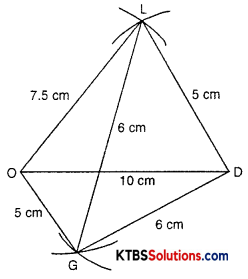(iii) Draw a rhombus BEND roughly.
Since the sides of a rhombus are equal.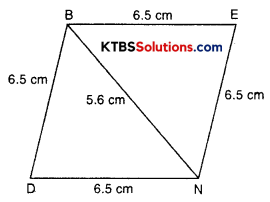Steps of construction:

1. Draw a line segment BN = 5.6 cm.
2. Draw an arc of radius 6.5 taking B as centre.
3. With the same radius draw an arc taking N as centre.
4. These arcs intersect at E.
5. BEN is a triangle.
6. Repeat the process to draw ∆BDN.
7. BEND is a required rhombus.